# Atomic force microscope

An atomic force microscope (AFM) measures the height of a surface by moving a cantilevered tip across a surface and measuring the motion of the cantilever as it moves across the surface. The AFM and similar instruments allow visualization of surface structure at the nanometer scale. In tapping mode the cantilever is vibrated, and the amplitude of vibration is controlled by feedback. In contact mode the cantilever is in contact with the sample, and its bending is controlled by feedback. In both cases control is actuated by a piezo element that controls the vertical position of the cantilever base (or the sample). The control system has a direct influence on picture quality and scanning rate.

## Contents

### AFM in contact mode

A simple model of an AFM in contact mode is obtained by assuming that the piezo crystal generates a forcebetween the masses and that there is a dampingin the spring. Let the positions of the center of the masses be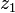and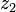. A momentum balance gives the following model for the system: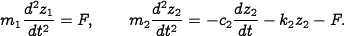Let the elongation of the piezo element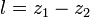be the control variable and the heightof the cantilever base be the output. Eliminating the variablein equations \eqref{eq:afm:trampoline} and substituting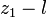forgives the model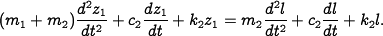The piezo stack can be modeled by a second-order system with undamped natural frequency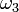and damping ratio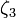. The dynamics are then described by the linear system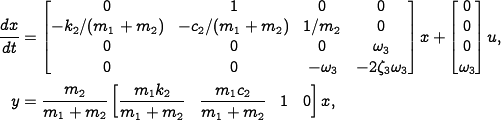where the input signal is the drive signal to the amplifier and the output is the elongation of the piezo.

MATLAB files:

### AFM in tapping mode

The dynamics of the tapping mode of an atomic force microscope are dominated by the damping of the cantilever vibrations and the system that averages the vibrations. Modeling the cantilever as a spring-mass system with low damping, we find that the amplitude of the vibrations decays as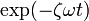, where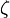is the damping ratio and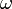is the undamped natural frequency of the cantilever. The cantilever dynamics can thus be modeled by the transfer function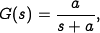where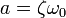. The averaging process can be modeled by the input/output relation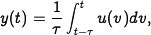where the averaging time is a multipleof the period of the oscillation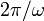. The dynamics of the piezo scanner can be neglected in the first approximation because they are typically much faster than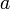. A simple model for the complete system is thus given by the transfer function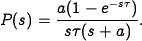MATLAB files:

• afmtap_integral.m - Nyquist and Bodep lots for AFM in tapping mode with integral control (Example 10.2)
• afmtap_zntuning.m - Ziegler-Nichols tuning for an AFM in tapping mode (Example 10.4)

### AFM nano positioner

The horizontal motion of an AFM is typically modeled as a spring-mass system with low damping. The normalized transfer function is given by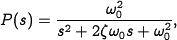where the damping ratio typically is a very small number, e.g.,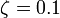.

MATLAB files:

• afmpos_integral.m - AFM nanopositioning systems with integral control (Example 9.9)